# High School Math : Graphing Exponential Functions

## Example Questions

### Example Question #1 : Graphing Exponential Functions

Find the-intercept(s) of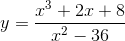.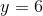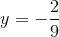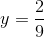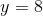This function does not cross the-axis.Explanation:

To find the-intercept, setin the equation and solve.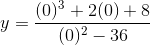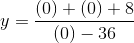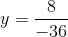### Example Question #2 : Graphing Exponential Functions

Find the-intercept(s) of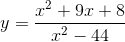.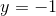and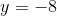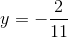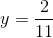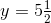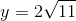Explanation:

To find the-intercept(s) of, set thevalue equal to zero and solve.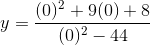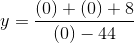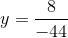### Example Question #3 : Graphing Exponential Functions

Find the-intercept(s) of.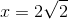and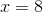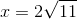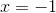and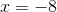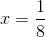andExplanation:

To find the-intercept(s) of, we need to set the numerator equal to zero and solve.

First, notice that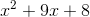can be factored into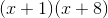. Now set that equal to zero: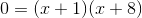.

Since we have two sets in parentheses, there are two separatevalues that can cause our equation to equal zero: one where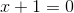and one where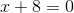.

Solve for each value:and.

Therefore there are two-interecpts:and.

### Example Question #4 : Graphing Exponential Functions

Find the-intercept(s) of.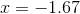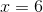or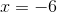The function does not cross the-axis.Explanation:

To find the-intercept(s) of, we need to set the numerator equal to zero.

That means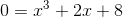.

The best way to solve for a funky equation like this is to graph it in your calculator and calculate the roots. The result is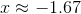.